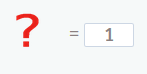Smartick is an advanced online program that teaches kids math and coding in only 15 min. a day

May13

# Smartick Logic Exercises with Operators

In today’s post, we are going to practice some Smartick logic exercises that parents ask us about fairly often: Operator exercises.

They are exercises that, at first glance, look complicated but once you understand them you see that they can become a game for children!

They form part of the logic exercises that stimulate mathematical talent intent on presenting the introduction to mathematics functions as a challenge.

We will see how to solve various examples.

### Example of operators in addition

To begin, let’s solve the following exercise:Here the symbolfunctions as an operator that relates M and D in a specific way.

If we look closely at the operation related to M and D, we see that in this case it is addition. So, in order to operate M and D, we need to add them.

Therefore, to operate 4 and 2, we need to add them as well: 4 + 2 = 6.

So:Do you see how simple it is?!

### Example of operators in subtraction

Now we will solve the next exercise:Now we have the symbolthat functions as an operator to relates E and C as a subtraction problem.

And in order to operate 8 and 4, we have to subtract 4 from 8, in other words, 8 – 4 = 4.

Then:I’m sure that you also found this one easy, right?

### Example of operators in subtraction and changing the order

And, lastly, we will solve the following exercise:Now we have this symbolwhich functions as an operator to relate T and F in a subtraction problem but changing the order of the letters. So, in order to operate T and F, we have to subtract T from F.

And to operate 8 and 9, we have to subtract 8 from 9 like this: 9 – 8 = 1.

Therefore:This exercise is a bit more complicated since you have to change the order of the letters but still simple, don’t you think?

I hope that these examples have helped you to understand operator exercises. Don’t forget to share this post if you liked it!

And, as you know, if you would like to practice more logic exercises, log on to Smartick and try it for free.